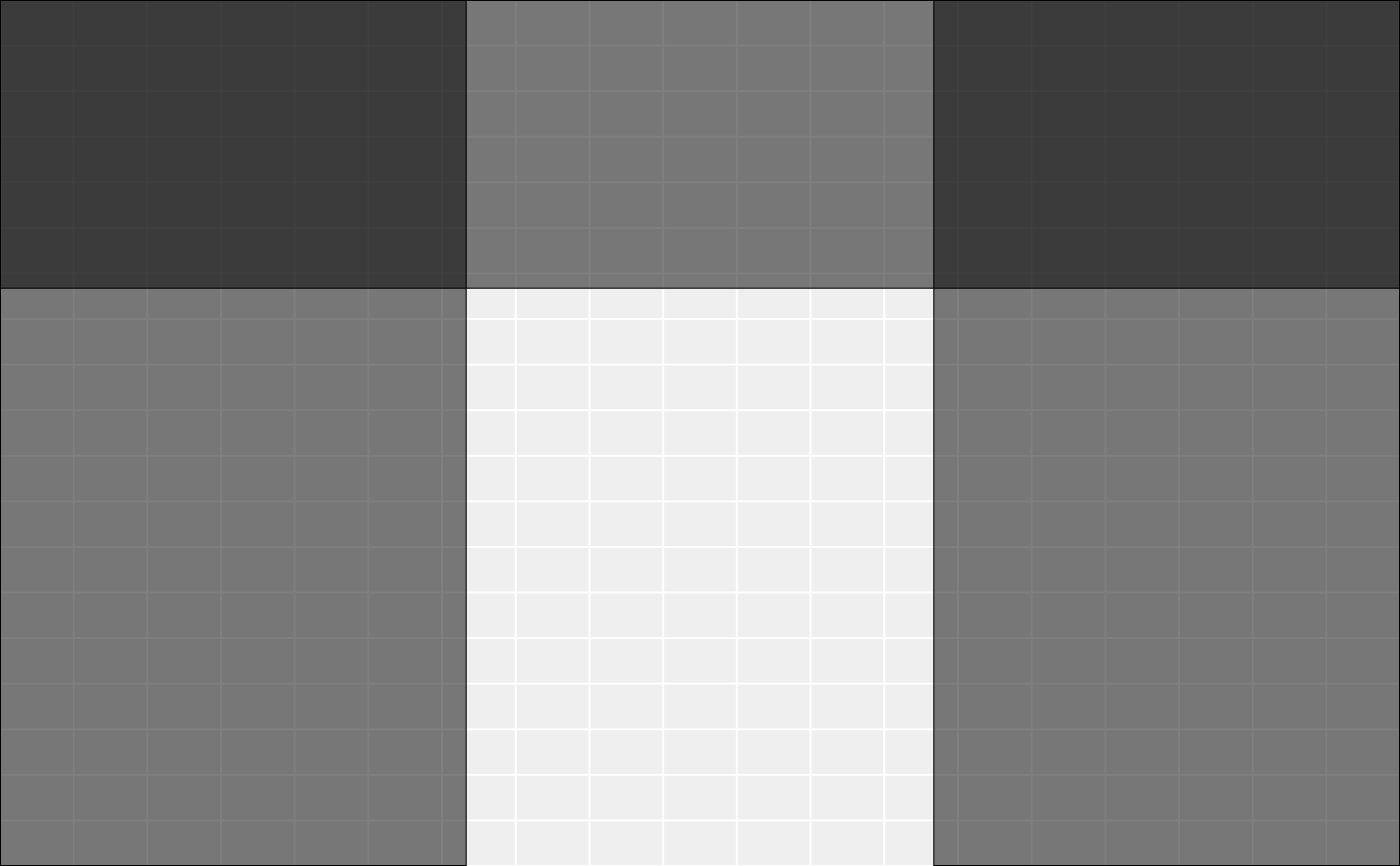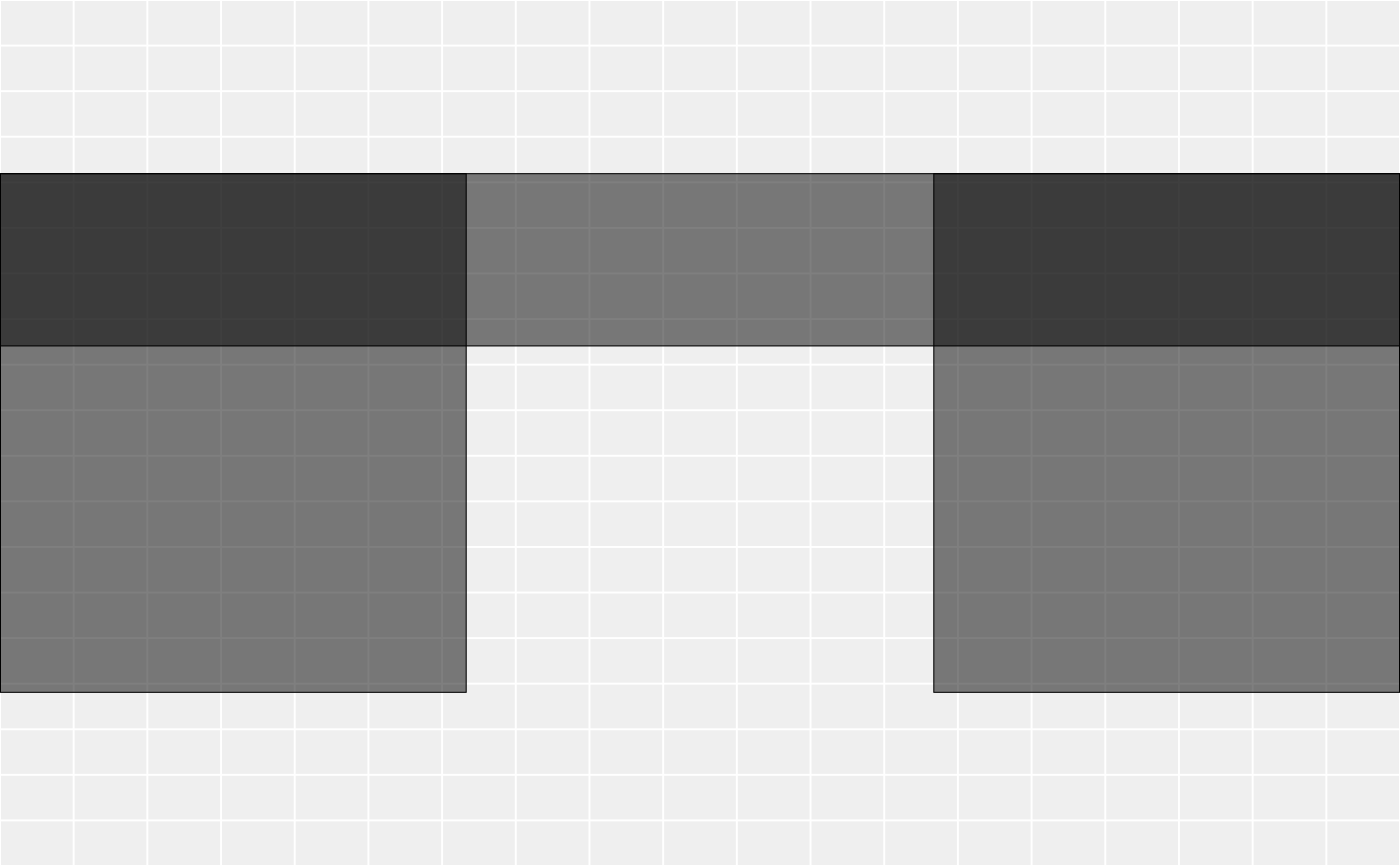Insert new rows in a gtable and adjust the grob placement accordingly. If rows are added in the middle of a grob spanning multiple rows, the grob will continue to span them all. If a row is added above or below a grob, the grob will not span the new row(s).

gtable_add_rows(x, heights, pos = -1)

## Arguments

x

a gtable() object

heights

a unit vector giving the heights of the new rows

pos

new row will be added below this position. Defaults to adding row on bottom. 0 adds on the top.

## Value

A gtable with the new rows added.

Other gtable manipulation: gtable_add_cols(), gtable_add_grob(), gtable_add_padding(), gtable_add_space, gtable_filter()

## Examples

library(grid)
rect <- rectGrob(gp = gpar(fill = "#00000080"))
tab <- gtable(unit(rep(1, 3), "null"), unit(rep(1, 3), "null"))
tab <- gtable_add_grob(tab, rect, t = 1, l = 1, r = 3)
tab <- gtable_add_grob(tab, rect, t = 1, b = 3, l = 1)
tab <- gtable_add_grob(tab, rect, t = 1, b = 3, l = 3)
dim(tab)
#>  3 3
plot(tab)# Grobs will continue to span over new rows if added in the middle
tab2 <- gtable_add_rows(tab, unit(1, "null"), 1)
dim(tab2)
#>  4 3
plot(tab2)# But not when added to top (0) or bottom (-1, the default)